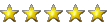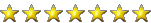# neural net in 9 python lines

#### vectoravtech

#####Code:
``````from numpy import exp, array, random, dot
training_set_inputs = array([[0, 0, 1], [1, 1, 1], [1, 0, 1], [0, 1, 1]])
training_set_outputs = array([[0, 1, 1, 0]]).T
random.seed(1)
synaptic_weights = 2 * random.random((3, 1)) - 1
for iteration in xrange(10000):
output = 1 / (1 + exp(-(dot(training_set_inputs, synaptic_weights))))
synaptic_weights += dot(training_set_inputs.T, (training_set_outputs - output) * output * (1 - output))
print 1 / (1 + exp(-(dot(array([1, 0, 0]), synaptic_weights))))``````
source https://medium.com/technology-invention-and-more/how-to-build-a-simple-neural-network-in-9-lines-of-python-code-cc8f23647ca1

not sure what I can train it on yet but heres the better version lol "longer, but more beautiful version of the source code"

74 lines

Code:
``````from numpy import exp, array, random, dot

class NeuralNetwork():
def __init__(self):
# Seed the random number generator, so it generates the same numbers
# every time the program runs.
random.seed(1)

# We model a single neuron, with 3 input connections and 1 output connection.
# We assign random weights to a 3 x 1 matrix, with values in the range -1 to 1
# and mean 0.
self.synaptic_weights = 2 * random.random((3, 1)) - 1

# The Sigmoid function, which describes an S shaped curve.
# We pass the weighted sum of the inputs through this function to
# normalise them between 0 and 1.
def __sigmoid(self, x):
return 1 / (1 + exp(-x))

# The derivative of the Sigmoid function.
# This is the gradient of the Sigmoid curve.
# It indicates how confident we are about the existing weight.
def __sigmoid_derivative(self, x):
return x * (1 - x)

# We train the neural network through a process of trial and error.
# Adjusting the synaptic weights each time.
def train(self, training_set_inputs, training_set_outputs, number_of_training_iterations):
for iteration in xrange(number_of_training_iterations):
# Pass the training set through our neural network (a single neuron).
output = self.think(training_set_inputs)

# Calculate the error (The difference between the desired output
# and the predicted output).
error = training_set_outputs - output

# Multiply the error by the input and again by the gradient of the Sigmoid curve.
# This means less confident weights are adjusted more.
# This means inputs, which are zero, do not cause changes to the weights.
adjustment = dot(training_set_inputs.T, error * self.__sigmoid_derivative(output))

# The neural network thinks.
def think(self, inputs):
# Pass inputs through our neural network (our single neuron).
return self.__sigmoid(dot(inputs, self.synaptic_weights))

if __name__ == "__main__":

#Intialise a single neuron neural network.
neural_network = NeuralNetwork()

print "Random starting synaptic weights: "
print neural_network.synaptic_weights

# The training set. We have 4 examples, each consisting of 3 input values
# and 1 output value.
training_set_inputs = array([[0, 0, 1], [1, 1, 1], [1, 0, 1], [0, 1, 1]])
training_set_outputs = array([[0, 1, 1, 0]]).T

# Train the neural network using a training set.
# Do it 10,000 times and make small adjustments each time.
neural_network.train(training_set_inputs, training_set_outputs, 10000)

print "New synaptic weights after training: "
print neural_network.synaptic_weights

# Test the neural network with a new situation.
print "Considering new situation [1, 0, 0] -> ?: "
print neural_network.think(array([1, 0, 0]))``````
run with
Code:
``python main.py``

Last edited:

#### R-T-B

#####Supporter
I'm counting more than 9 python code lines, but ok.